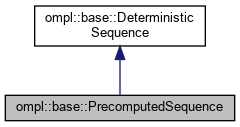ompl::base::PrecomputedSequence Class Reference

General realization for a sampler of precomputed sequences or sets. More...

#include <ompl/base/samplers/deterministic/PrecomputedSequence.h>

Inheritance diagram for ompl::base::PrecomputedSequence:[legend]

## Public Member Functions

PrecomputedSequence (std::string path, unsigned int dimensions, bool shuffle=false, size_t max_samples=0)
Constructor, requires the path of file containing the precomputed samples formated with one sample per line, with the dimensions separated by whitespace. Optionally the order of the samples can be randomized (which removes deterministim, if not all samples are used), max_samples optionally limits the number of samples to be read from the file (default 0 means all samples are read), scale_factor optinonally scales the the samples (if the precomputed samplesets are e.g. in range 0,1.

std::vector< double > sample () override
Returns the next sample, loops if there are no more precomputed samples. The range of the samples depends on the precomputed set of samples.Public Member Functions inherited from ompl::base::DeterministicSequence
DeterministicSequence (unsigned int dimensions)
Constructor.Public Attributes inherited from ompl::base::DeterministicSequence